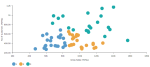# MLLIB.BICLUSTER(imputer, n_clusters, seed, columns)

Bisecting K-means is a kind of hierarchical clustering using divisive (top-down approach), where all observations start in one cluster, and splits are performed recursively as it moves down the hierarchy. The splits are done with regular K-means with K = 2 on a cluster with highest SSE (sum of squared errors). The algorithm is executed with 20 iterations to split clusters.

Bisecting K-means can often be much faster than regular K-means, but it will generally produce a different clustering.

###### Parameters
• imputer – strategy for dealing with null values:

• 0 – Replace null values with ‘0'

• 1 – Assign null values to a designated ‘-1' cluster

• number_of_clusters – Number of clusters which the algorithm should find, integer.

• seed – Random seed, integer.

• columns – Dataset columns or custom calculations.

Example: MLLIB.BICLUSTER(0, 3, 555, sum([Gross Sales]), sum([No of customers])) used as a calculation for the Color field of the Scatterplot visualization.###### Input data
• Size of input data is not limited.
• Without missing values.
• Character variables are transformed to numeric with label encoding.
###### Result
• Column of integer values starting with 0, where each number corresponds to a cluster assigned to each record (row) by the Bisecting K-means algorithm.
###### Key usage points
• Less sensitivity to initialization than regular K-means.
• Tends to produce clusters of similar sizes, where K-means often produces null clusters when k is large.
• Lower computational time.
• Use it when you want to avoid convergence in local minimum.
###### Drawbacks
• If the number of clusters is not selected properly, it will cause a large deviation between the results and ideal clustering results.

For the whole list of algorithms, see Data science built-in algorithms.